# Stiefel number

(diff) ← Older revision | Latest revision (diff) | Newer revision → (diff)

A characteristic number of a closed manifold taking values in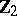, the integers modulo 2. Let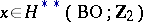be an arbitrary stable characteristic class, and letbe a closed manifold. The residue modulo 2 defined byis called the Stiefel number (or Stiefel–Whitney number) ofcorresponding to the class. Here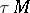is the tangent bundle of, and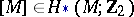is the fundamental class. For-dimensional manifolds, the Stiefel number depends only on the-th homogeneous component of the class. The group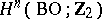is isomorphic to a vector space over the fieldwhose basis is in one-to-one correspondence with the set of all partitions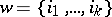of the number, i.e. tuples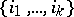of non-negative integers such that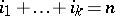. The classeswould be a natural choice for a basis of. Thus, to characterize a manifold by its Stiefel numbers it is sufficient to consider the classes, whereis a partition of the dimension of the manifold.

Bordant manifolds have the same Stiefel numbers, since each characteristic classdetermines a homomorphism, where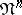is the group of classes of bordant non-oriented-dimensional manifolds. If for two closed manifolds,the equalityholds for all partitionsof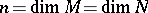, then the manifoldsandare bordant (Thom's theorem).

Letbe the vector space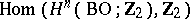over the field. Letbe the basis indual to the basis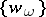in,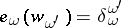, hereare partitions of; and let a mappingbe defined by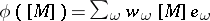. The mappingis monomorphic, and for a complete description of the groupby the Stiefel numbers it is necessary to find its image. This problem is analogous to the Milnor–Hirzebruch problem for Chern classes (cf. Chern class). For a closed manifold, let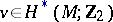be the so-called Wu class, uniquely defined by, which should hold for all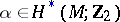. Then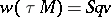, whereis the tangent bundle to(Wu's theorem).

This theorem implies that the Wu class can be defined as a characteristic class: Let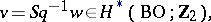whereis the complete Stiefel–Whitney class andis the cohomology operation inverse to the complete Steenrod square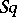. Let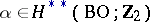be an arbitrary characteristic class. Then for any closed manifold the numbersand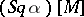coincide. Thus, an element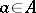,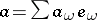can be in the image of the mappingonly if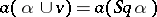holds for all. For a homomorphism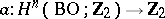there exists a manifold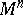such that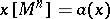for allif and only iffor all(Dold's theorem).

For references, see Stiefel–Whitney class.

As is customary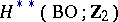denotes the direct product of the cohomology groupsof the classifying space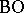, while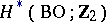is the direct sum.